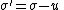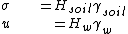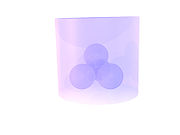xEffective stressEncyclopedia
Karl von Terzaghi
Karl von Terzaghi
Karl von Terzaghi was an Austrian civil engineer and geologist, called the father of soil mechanics.-Early life:...

first proposed the relationship for effective stress in 1936. http://fbe.uwe.ac.uk/public/geocal/SoilMech/stresses/stresses.htm For him, the term ‘effective’ meant the calculated stress
Stress (physics)
In continuum mechanics, stress is a measure of the internal forces acting within a deformable body. Quantitatively, it is a measure of the average force per unit area of a surface within the body on which internal forces act. These internal forces are a reaction to external forces applied on the body...

that was effective in moving soil, or causing displacements. It represents the average stress carried by the soil skeleton.

Effective stress (σ') acting on a soil is calculated from two parameters, total stress (σ) and pore water pressure
Pore water pressure
Pore water pressure refers to the pressure of groundwater held within a soil or rock, in gaps between particles . Pore water pressures in below the phreatic level are measured in piezometers...

(u) according to:Typically, for simple examplesMuch like the concept of stress itself, the formula is a construct, for the easier visualization of forces acting on a soil mass, especially simple analysis models for slope stability
Slope stability
The field of slope stability encompasses the analysis of static and dynamic stability of slopes of earth and rock-fill dams, slopes of other types of embankments, excavated slopes, and natural slopes in soil and soft rock...

, involving a slip plane.http://www.dur.ac.uk/~des0www4/cal/slopes/page4.htm With these models, it is important to know the total weight of the soil above (including water), and the pore water pressure within the slip plane, assuming it is acting as a confined layer.

However, the formula becomes confusing when considering the true behaviour of the soil particles under different measurable conditions, since none of the parameters are actually independent actors on the particles.Consider a grouping of round quartz
Quartz
Quartz is the second-most-abundant mineral in the Earth's continental crust, after feldspar. It is made up of a continuous framework of SiO4 silicon–oxygen tetrahedra, with each oxygen being shared between two tetrahedra, giving an overall formula SiO2. There are many different varieties of quartz,...

sand grains, piled loosely, in a classic ‘cannonball’ arrangement. As can be seen, there is a contact stress where the spheres actually touch. Pile on more spheres and the contact stresses increase, to the point of causing frictional instability (dynamic friction
Friction
Friction is the force resisting the relative motion of solid surfaces, fluid layers, and/or material elements sliding against each other. There are several types of friction:...

), and perhaps failure. The independent parameter affecting the contacts (both normal and shear) is the force of the spheres above. This can be calculated by using the overall average density
Density
The mass density or density of a material is defined as its mass per unit volume. The symbol most often used for density is ρ . In some cases , density is also defined as its weight per unit volume; although, this quantity is more properly called specific weight...

of the spheres and the height of spheres above.If we then have these spheres in a beaker
Beaker
Beaker may refer to:* Beaker , a beverage container* Beaker , or "laboratory beaker", a glass object used for holding fluids and chemicals in a laboratory setting.* Beaker , a prehistoric drinking vessel...

and add some water, they will begin to float a little depending on their density (buoyancy
Buoyancy
In physics, buoyancy is a force exerted by a fluid that opposes an object's weight. In a column of fluid, pressure increases with depth as a result of the weight of the overlying fluid. Thus a column of fluid, or an object submerged in the fluid, experiences greater pressure at the bottom of the...

). With natural soil materials, the effect can be significant, as anyone who has lifted a large rock out of a lake can attest. The contact stress on the spheres decreases as the beaker is filled to the top of the spheres, but then nothing changes if more water is added. Although the water pressure between the spheres (pore water pressure) is increasing, the effective stress remains the same, because the concept of 'total stress' includes the weight of all the water above. This is where the equation can become confusing, and the effective stress can be calculated using the buoyant density of the spheres (soil), and the height of the soil above.The concept of effective stress truly becomes interesting when dealing with non-hydrostatic pore water pressure. Under the conditions of a pore pressure gradient, the ground water flows, according to the permeability equation (Darcy's law
Darcy's law
Darcy's law is a phenomenologically derived constitutive equation that describes the flow of a fluid through a porous medium. The law was formulated by Henry Darcy based on the results of experiments on the flow of water through beds of sand...

). Using our spheres as a model, this is the same as injecting (or withdrawing) water between the spheres. If water is being injected, the seepage force acts to separate the spheres and reduces the effective stress. Thus, the soil mass becomes weaker. If water is being withdrawn, the spheres are forced together and the effective stress increases. http://fbe.uwe.ac.uk/public/geocal/SoilMech/water/water.htm

Two extremes of this effect are quicksand
Quicksand
Quicksand is a colloid hydrogel consisting of fine granular matter , clay, and water.Water circulation underground can focus in an area with the optimal mixture of fine sands and other materials such as clay. The water moves up and then down slowly in a convection-like manner throughout a column...

, where the groundwater gradient and seepage force act against gravity; and the 'sandcastle effect', http://home.tu-clausthal.de/~pcdj/publ/PRL96_058301.pdf where the water drainage and capillary action act to strengthen the sand. As well, effective stress plays an important role in slope stability
Slope stability
The field of slope stability encompasses the analysis of static and dynamic stability of slopes of earth and rock-fill dams, slopes of other types of embankments, excavated slopes, and natural slopes in soil and soft rock...

, and other geotechnical engineering
Geotechnical engineering
Geotechnical engineering is the branch of civil engineering concerned with the engineering behavior of earth materials. Geotechnical engineering is important in civil engineering, but is also used by military, mining, petroleum, or any other engineering concerned with construction on or in the ground...

and engineering geology
Engineering geology
Engineering geology is the application of the geologic sciences to engineering practice for the purpose of assuring that the geologic factors affecting the location, design, construction, operation and maintenance of engineering works are recognized and adequately provided for...

problems, such as groundwater-related subsidence
Groundwater-related subsidence
Groundwater-related subsidence is the subsidence of land resulting from groundwater extraction, and a major problem in the developing world as major metropolises swell without adequate regulation and enforcement, as well as a being a common problem in the developed world...

.